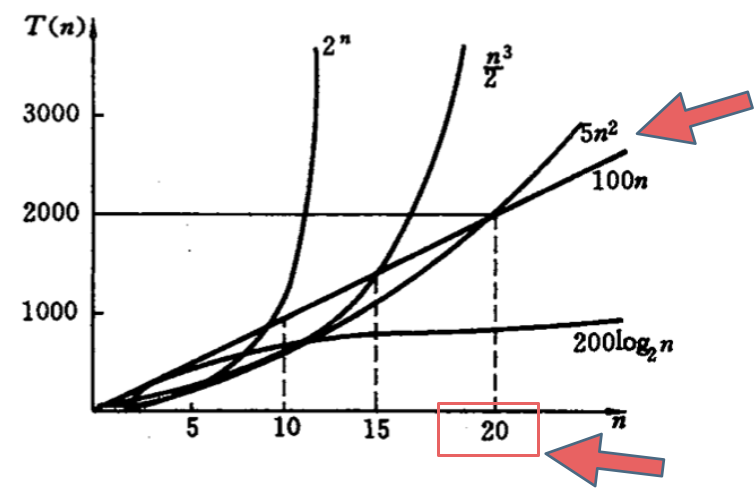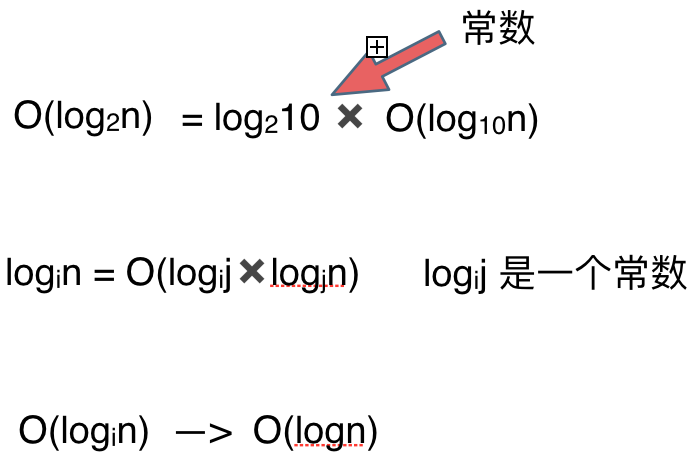• 究竟什么是时间复杂度
• 什么是大O
• 不同数据规模的差异
• 复杂表达式的化简
• \$O(\log n)\$中的log是以什么为底？
• 举一个例子

# 不同数据规模的差异O(1)常数阶 < \$O(\log n)\$对数阶 < \$O(n)\$线性阶 < \$O(n^2)\$平方阶 < \$O(n^3)\$立方阶 < \$O(2^n)\$指数阶

# 复杂表达式的化简

O(2*n^2 + 10*n + 1000)
1

O(2*n^2 + 10*n)
1

O(n^2 + n)
1

O(n^2)
1

O(n^2)
1

# \$O(\log n)\$中的log是以什么为底？# 总结

@2021-2022 代码随想录 版权所有 粤ICP备19156078号-3